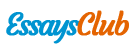# Financial Markets and Institutions Problem Set

Autor:   •  October 18, 2017  •  731 Words (3 Pages)  •  240 Views

Page 1 of 3

...

+ (.05)*(24) = .25 = 25%

What is the standard deviation of the investment return?

Variance = (.95)*(-1-.25)2 + (.05)*(24-.25)2

StDev = (variance)1/2

StDev = 5.45%

Question 4 – Development of two drugs

Suppose there is another drug compound B that has potential to slow the growth of cancer cells in the breast. It has exactly the same payoff as compound A. The successes of the two drug developments are independent.

Drug B initial investment 100

Scenario Probability Payoff Returns

F 95% 0 -1

P 5% 2500 24

What is the probability of both drugs passing FDA approvals?

(.05)*(.05) = .0025 = .25%

What about the probability of both drugs failing FDA approvals?

(.95)*(.95) = .9025 = 90.25%

What is the probability of drug A passing FDA approval and drug B failing FDA approval? Or vice versa?

(.05)*(.95) = .0475 = 4.75%

What about the probability of at least one drug passing FDA approvals?

1 - .9025 = .0975 = 9.75%

Suppose an investor invest \$100 million into the two compounds, allocating half the money to each compound. Fill in the probabilities, payoffs and returns of the following scenarios.

Drugs A and B initial investment 100 (half in each)

Scenario Probability Payoff Returns

FF 90.25% 0 -1

PF 4.75% 1250 11.5

FP 4.75% 1250 11.5

PP .25% 2500 24

What is the expected return of this investment?

(1/2)(.25) + (1/2)*(.25) = (.5)*(.25)*(2) = .25 = 25%

What is the standard deviation of the investment return?

Variance = (1/2)2 * (5.45)2 * (2)

StDev = (variance)1/2 = 3.85%

Question 5 – Development of 100 drugs

Suppose there are 100 drugs that are being invested. Each drug has exactly the same payoff as compound A. The successes of the all drug developments are independent. Suppose the investor invest \$100 million into the 100 compounds, allocating \$1 million to each compound.

What is the expected return of this investment?

(1/100)*(.25)*(100) = .25 = 25%

What is the probability of at least one drug passing FDA approvals?

1 – (.95)100 = .9941 = 99.41%

What is the standard deviation of the investment return?

Variance = (1/100)2 * (5.45)2 * (100)

StDev = (variance)1/2 = .545%

...